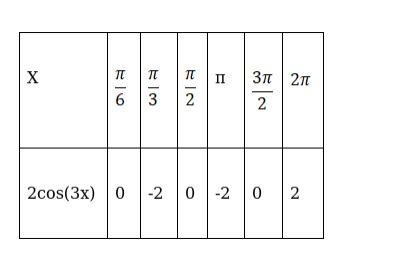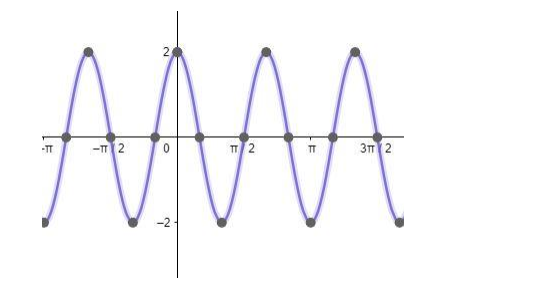# Draw the graph of each of the following functions:`
Question:

Draw the graph of each of the following functions:

2cos 3x

Solution:

To draw the graph of the curve 2cos(3x) assume some standard angle measures which will help in locating the points and drawing the curve.Therefore, the graph of curve 2cos(3x) can be drawn asHere, the amplitude and frequency of the function cos(x) is increased by 2 and 3 times respectively.